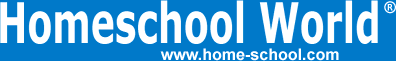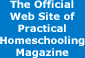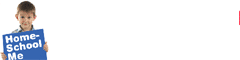Home Curriculum Catalog Articles Contests Events Groups Forum News ContactGetting Started Preschool/K Elementary Middle School High School College & Career Prep Special Needs Group Activities Research FAQs# The Equal Sign - Symbol, Name, Meaning

By Bob Hazen
Printed in Practical Homeschooling #29, 1999.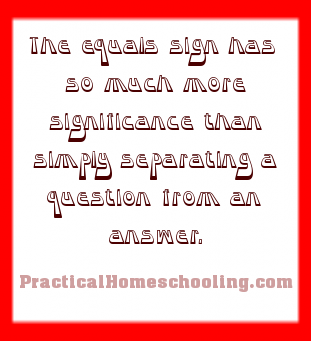What does "=" mean?What does the sign of "=" mean? Of course, the name of the symbol is "the equal sign," or "equals," and it is valuable to know how to verbalize or say the proper words for a symbol such as this. But the question is, "What does the equal sign mean?" For this question we must add the caveat, "You cannot use the name of the symbol to tell what the symbol means."

Without this caveat, children often try to explain what the equal sign means by saying something to the effect, "Well, the equal sign means that things are equal." To show the importance of not using the same word to explain what a given word means, I take the relatively unknown English word gloaming and explain what it means by using that very same word: "Well, gloaming is when it's gloaming, so when it's gloaming, that's when it's gloaming, so that's what gloaming means."

After this self-referential go-around, I then ask the children, "Do you now have any better idea of what gloaming means?" Of course, they shake their heads "no," realizing that it is clearer and more precise to use other words to tell what a particular word means.

So, to reiterate my point, my next question is, "Without using the word 'equal,' what does the equal sign mean?" Here are some common answers, along with my responses: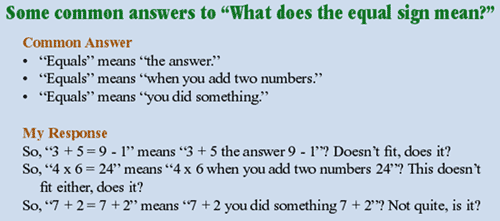By now, I've really got their attention. Sometimes, a child will correctly identify one of the proper meanings of the equal sign, but if not, after letting children try for a while, I will give the following multi-step explanation:

• "Equals" means "is," so "3 + 5 = 8" means "3 + 5 is 8," while "7 + 2 = 7 + 2" means simply "7 + 2 is 7 + 2."

• Sometimes, we can also say that "equals" means "is the same value as," such as when we say correctly that "1 dollar = 4 quarters." It is not quite correct to say that "1 dollar is 4 quarters," since it is not true that 1 piece of paper is 4 round pieces of metal. This is where we have to bring in an alternate meaning of the equal sign, and in this example, "1 dollar is the same value as 4 quarters" is a better definition of what "equals" means.

• In summary, the equal sign means either simply is or simply is the same value as. That is all the equal sign means - nothing more, and literally, nothing less.

The value of having an accurate sense of the what the equal sign means is important at every level of mathematics. It is important at the elementary level for several reasons. First, accuracy and truthfulness are important, period. Second, accuracy and truthfulness are especially important when beginning something, since proper understanding and precise perception are the stage for everything that follows. Inaccurate or only partially true definitions might work for a while, but they eventually stop working, while also wreaking havoc along the way. Third, an accurate understanding of the equal sign sets students up for success when they encounter more abstract mathematical concepts and procedures in upper elementary and junior high school math. Math in grades 5-8 - especially algebra and pre-algebra - are filled with examples of mathematical statements that can be confusing if the proper meaning of the equal sign is not in place. In each of the following examples, it is especially helpful to stay away from any sense that the equal sign means "the answer" or "I did something" or "add two numbers." These examples use the equal sign to emphasize the simple fact that two quantities have the same value:

• A = L x W [area of a rectangle is the same value as the rectangle's length times the rectangle's width];

• 3x(5+7) = 3x5 + 3x7 [the Distributive Property of Multiplication over Addition: first adding two numbers 5 and 7 and then multiplying the result by 3 is the same value as first multiplying 3 times 5 and 3 times 7 and then adding those results];

• 4x + 26 = 6x + 10 [a simple equation in one variable, stating that 4 of an unknown number added to 26 is the same value as 6 of that same unknown number added to 10];

• c2 = a2 + b2 [the square of the hypotenuse c of a right triangle is the same value as the square of the side a added to the square of side b].

If students erroneously think that the equal sign means "I did something," for example, then what does the formula for area ("A = L x W") mean? "Area I did something length times width?" Children will correctly realize that doesn't make sense, and they may come away from that encounter also sensing that math just doesn't make sense - all because of an erroneous understanding of what the equal sign means.

In pre-algebra and algebra, students need to understand the steps of solving a simple equation in one variable. This important skill is often made unnecessarily more difficult by incorrect and misleading interpretations of the equal sign. The contrast between an improper and proper understanding of these steps is examined in Chart 2, where the equal sign first is erroneously interpreted in the middle column to mean "I did something" or "is the answer to." In the third column, the equal sign is correctly interpreted to mean "is the same value as."

This chart should help readers see at least one reason why some children "just don't get math." The erroneous interpretation of what the equal sign means is far from being one small thing that's no big deal. An inaccurate idea of this most basic of mathematical symbols can create confusion that unsettles our children for years and years. Eventually, children often implicitly or unconsciously adapt to more or less an accurate idea of what the equal sign means. But the damage that has been done in the meantime often will have already shaped and molded a child's attitude about math and that child's perception of both mathematical concepts and one's own ability.

Accuracy and truthfulness are important, period. Let's be accurate and truthful with our children. Let's equip them with the accurate tools and truthful understandings they will need to help them be better in whatever God has for them in mathematics.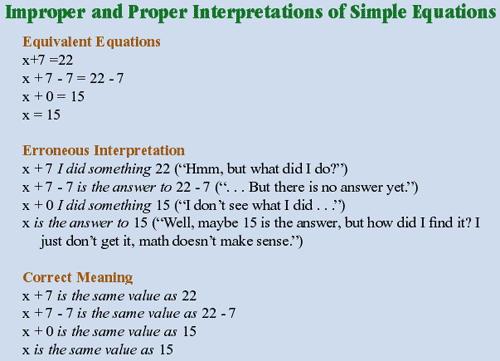Terms of Use   Privacy Policy Copyright ©1993-2023 Home Life, Inc.